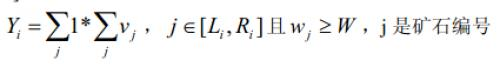# P1314 聪明的质监员

• 5.6K通过
• 21.4K提交
• 题目提供者 CCF_NOI
• 评测方式 云端评测
• 标签 二分答案 前缀和 数论,数学 NOIp提高组 2011 高性能
• 难度 提高+/省选-
• 时空限制 1000ms / 128MB
• 提示：收藏到任务计划后，可在首页查看。

## 题目描述

小T 是一名质量监督员，最近负责检验一批矿产的质量。这批矿产共有 $n$ 个矿石，从 $1$到$n$ 逐一编号，每个矿石都有自己的重量 $w_i$ 以及价值$v_i$ 。检验矿产的流程是：

1 、给定$m$个区间$[L_i,R_i]$；

2 、选出一个参数$W$；

3 、对于一个区间$[L_i,R_i]$，计算矿石在这个区间上的检验值$Y_i$：这批矿产的检验结果$Y$ 为各个区间的检验值之和。即：$Y_1+Y_2...+Y_m$

若这批矿产的检验结果与所给标准值$S$ 相差太多，就需要再去检验另一批矿产。小T不想费时间去检验另一批矿产，所以他想通过调整参数W 的值，让检验结果尽可能的靠近标准值$S$，即使得$S-Y$ 的绝对值最小。请你帮忙求出这个最小值。

## 输入输出格式

输入格式：

第一行包含三个整数$n,m,S$，分别表示矿石的个数、区间的个数和标准值。

接下来的$n$行，每行$2$个整数，中间用空格隔开，第$i+1$行表示$i$号矿石的重量$w_i$和价值$v_i$。

接下来的$m$ 行，表示区间，每行$2$ 个整数，中间用空格隔开，第$i+n+1$ 行表示区间$[L_i,R_i]$的两个端点$L_i$ 和$R_i$。注意：不同区间可能重合或相互重叠。

输出格式：

一个整数，表示所求的最小值。

## 输入输出样例

输入样例#1： 复制
5 3 15
1 5
2 5
3 5
4 5
5 5
1 5
2 4
3 3 
输出样例#1： 复制
10


## 说明

【输入输出样例说明】

当$W$选$4$的时候，三个区间上检验值分别为$20,5 ,0$ ，这批矿产的检验结果为 $25$，此时与标准值$S$相差最小为$10$。

【数据范围】

对于$10\%$的数据，有 $1 ≤n ,m≤10$；

对于$30\%$的数据，有 $1 ≤n ,m≤500$ ；

对于$50\%$的数据，有$1 ≤n ,m≤5,000$；

对于$70\%$ 的数据，有 $1 ≤n ,m≤10,000$ ；

对于$100\%$的数据，有$1 ≤n ,m≤200,000,0 < w_i,v_i≤10^6,0 < S≤10^{12},1 ≤L_i ≤R_i ≤n$ 。

提示
标程仅供做题后或实在无思路时参考。
请自觉、自律地使用该功能并请对自己的学习负责。
如果发现恶意抄袭标程，将按照I类违反进行处理。